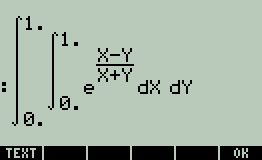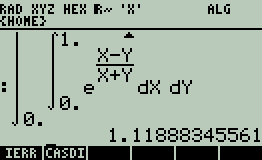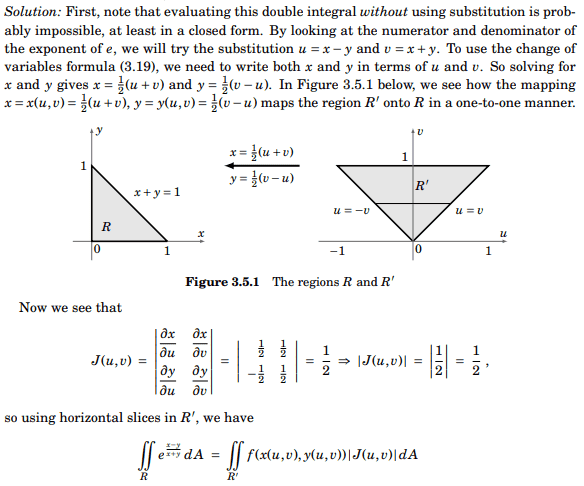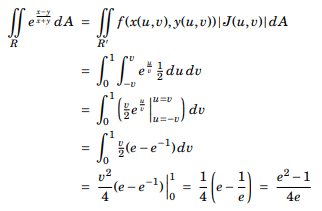# HP 50g calculator's answer is correct or author's answer is correct?

• WMDhamnekar

#### WMDhamnekar

MHB
Summary: Evaluate ##\displaystyle\iint\limits_R e^{\frac{x-y}{x+y}} dA ## where ##R {(x,y): x \geq 0, y \geq 0, x+y \leq 1}##

Author has given the answer to this question as ## \frac{e^2 -1}{4e} =0.587600596824 ## But hp 50g pc emulator gave the answer after more than 11 minutes of time 1.11888345561.Now, How to decide which answer is correct?

$$\int_{x=0}^1\int_{y=0}^{1 - x} e^{\frac{x-y}{x+y}}dy dx$$
••